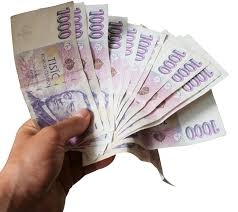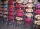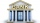# A man

A man spends 5/9 of his money on rent, and 5/16 of the remainder on electricity. If the final balance remaining is 550 find how much was spent on rent

Correct result:

x =  440

#### Solution:We would be pleased if you find an error in the word problem, spelling mistakes, or inaccuracies and send it to us. Thank you!Tips to related online calculators
Need help calculate sum, simplify or multiply fractions? Try our fraction calculator.
Do you have a linear equation or system of equations and looking for its solution? Or do you have quadratic equation?

## Next similar math problems:

• Mixed2improperWrite the mixed number as an improper fraction. 166 2/3
• Equation 29Solve next equation: 2 ( 2x + 3 ) = 8 ( 1 - x) -5 ( x -2 )
• Write 2Write 791 thousandths as fraction in expanded form.
• Simple equation 8Solve the following equation: 36=-(1+7x)-6(-7-x)
• Simple equation 9Solve the following equation: -8y+5=-9y+9
• Simple equationSolve the following simple equation: 2. (4x + 3) = 2-5. (1-x)
• In fractionsAn ant climbs 2/5 of the pole on the first hour and climbs 1/4 of the pole on the next hour. What part of the pole does the ant climb in two hours?
• Unknown numberI think the number - its sixth is 3 smaller than its third.
• Unknown number 6Determine the unknown number, which is by 1.5 greater than its fourth.
• One thirdIf 3/5 is 360, how much is 1/3?
• EquationFind x: x + 1/2 = 1/3
• Passenger boatTwo-fifths of the passengers in the passenger boat were boys. 1/3 of them were girls and the rest were adult. If there were 60 passengers in the boat, how many more boys than adult were there?
• Equation 15Solve equation with variables on both sides:
• Trees3/5 trees are apples, cherries are 1/3. 5 trees are pear. How many is the total number of trees?Cris had 15000 . He spent 1/3 of his money on a table and the remaining on 5 similar chairs that cost the same. How much did one chair cost?Peter spends 1/5 of his earnings on his rent and he saves 2/7. What fraction of his earnings is left?Lita had some money. She spent 1/2 of it on a hand bag and 1/3 of the remainder of the blouse. She had 260.00. How much money did she have at start?